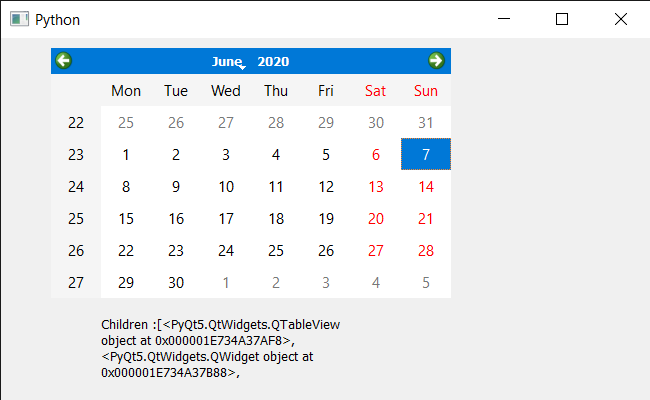# PyQt5 QCalendarWidget – Getting children using the type

• Last Updated : 29 Sep, 2021

In this article, we will see how we can find the children of QCalendarWidget using the type of the child/children. Calendar is not a stand alone widget it consists of small widgets that are called child for example the dates and spin box for year and drop down menu for the month collectively make the calendar. We can find the child using type with the help of findChild method but this method only return single widget therefore if there is more than one child with similar type then problem occurs.

In order to do this we will use findChildren method with the QCalendarWidget object.
Syntax : calendar.findChildren(type)
Argument : It takes type of the child argument
Return : It return list

Below is the implementation

## Python3

 `# importing libraries``from` `PyQt5.QtWidgets ``import` `*``from` `PyQt5 ``import` `QtCore, QtGui``from` `PyQt5.QtGui ``import` `*``from` `PyQt5.QtCore ``import` `*``import` `sys`  `class` `Window(QMainWindow):` `    ``def` `__init__(``self``):``        ``super``().__init__()` `        ``# setting title``        ``self``.setWindowTitle(``"Python "``)` `        ``# setting geometry``        ``self``.setGeometry(``100``, ``100``, ``650``, ``400``)` `        ``# calling method``        ``self``.UiComponents()` `        ``# showing all the widgets``        ``self``.show()` `    ``# method for components``    ``def` `UiComponents(``self``):` `        ``# creating a QCalendarWidget object``        ``self``.calendar ``=` `QCalendarWidget(``self``)` `        ``# setting geometry to the calendar``        ``self``.calendar.setGeometry(``50``, ``10``, ``400``, ``250``)` `        ``# setting cursor``        ``self``.calendar.setCursor(Qt.PointingHandCursor)` `        ``# creating label to show the properties``        ``self``.label ``=` `QLabel(``self``)` `        ``# setting geometry to the label``        ``self``.label.setGeometry(``100``, ``280``, ``250``, ``60``)` `        ``# making label multi line``        ``self``.label.setWordWrap(``True``)` `        ``# getting children through type``        ``value ``=` `self``.calendar.findChildren(QWidget)` `        ``# setting text to the label``        ``self``.label.setText(``"Children :"` `+` `str``(value))`   `# create pyqt5 app``App ``=` `QApplication(sys.argv)` `# create the instance of our Window``window ``=` `Window()` `# start the app``sys.exit(App.``exec``())`

Output :My Personal Notes arrow_drop_up